## Variance

Variance is an average of square deviation from the arithmetic mean.

Other name: Average square deviation

Formula: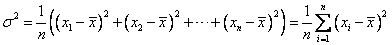Example: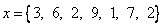Special for only two positive numbers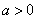and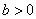, we have Variance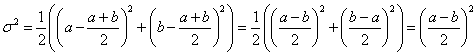Example: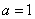,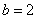, then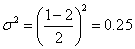To give you better understanding on the computation of variance, you may want to try with your own input data using the interactive Variance program below. To use the program, simply type your input data (only numbers input are accepted) in the box below, separated by comma. Then click "Get Variance" button.